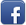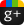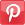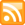# how can we add data from dynamic fields to mysql db in php

Posted on 16th Feb 2014 07:03 pm by admin

i have a script which i found on the internet. i modify that script according to my needs. what is in that script is there are three form fields with two buttons. one button is "Give me more fields" clicking on this button will give you more fields. and second button is submit so the data goes to server side and will be added to db. the problem is when i click give me more fields it gives me three more fields which is right but when i fill all these fields and click submit button it adds to the db but the data in the first three fields adds in the one row and the other three fields data adds in separate row which is not fine for me. so how can i do this so all the data will be added to only one row.

here is js code
Code: [Select]var counter = 0;
//Start a counter. Yes, at 0
counter++;
// I find it easier to start the incrementing of the counter here.
newFields.id = '';
newFields.style.display = 'block';
var newField = newFields.childNodes;
for (var i=0;i<newField.length;i++) {
var theName = newField[i].name
if (theName)
newField[i].name = theName + counter;
// This will change the 'name' field by adding an auto incrementing number at the end. This is important.
}
// Inside the getElementById brackets is the name of the div class you will use.
insertHere.parentNode.insertBefore(newFields,insertHere);
}
here is form
Code: [Select]<form name="addAQualification" action="<?php echo \$_SERVER['PHP_SELF']; ?>" method="post" enctype="multipart/form-data">
<fieldset>
<div id="phone">
Degree:
<input type="text" name="degree_0" value="" />

<input type="text" name="cgpa_0" value="" />

Institute:
<input type="text" name="institute_0" value="" />

</div>
Degree:
<input type="text" name="degree_" value="" />

<input type="text" name="cgpa_" value="" />

Institute:
<input type="text" name="institute_" value="" />

</div>

<input type="submit" name="submit" value="submit" />
</fieldset>
</form>and here is php
Code: [Select]<?php
if(isset(\$_POST['submit']))
//This checks to make sure submit was clicked
{
echo "You clicked submit!
";
";
echo "
";
if (\$_POST['cgpa_0'])
//This checks that the proper field has data
{
\$continue = FALSE;
\$i = 0;
while (\$continue == FALSE)
{
if (isset(\$_POST['degree_'.\$i]))
//This looks for an entry after 0 and increments
{
echo \$_POST['degree_'.\$i] . " = " . \$_POST['cgpa_'.\$i] . "
";
//Echoing the data
\$degree1 = \$_POST['degree_'.\$i];
\$cgpa1 = \$_POST['cgpa_'.\$i];
\$institute1 = \$_POST['institute_'.\$i];
\$db = mysql_connect("localhost");
mysql_select_db("test", \$db);
\$query = "INSERT INTO cv
(
degree1,
cgpa1,
institute1
)
VALUES
(
'\$degree1',
'\$cgpa1',
'\$institute1'
)";
\$result = mysql_query(\$query);
//The four lines above is the example on how the data would be put into a MySQL database. It's not used here
}
else
{
\$continue = TRUE;
}
\$i++;
}
}
}
?>

75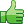48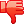##### Other forums

Comprar Replica relojes en línea, Rolex relojes clásicos de réplica en venta, falso Rolex y Omega relojes en venta
[b][url=http://www.watchdesigner.cn/es/]réplicas de relojes suizos aaa +[/url][/b] [b][url=http://w

Require select-option functionality in Module Pool Programming
Hi Gurus,

I am doing a development by Module Pool on Warehouse Management. For that devel

??????? ???? Taghuer
[b]швейцарские часы реплики AAA +[/b] [b][url=http://www.menswatches.cc/ru/]ш

Java API in PHP?
I have an application that we use internally here at the office.

The software company provide

rdns with php
I am trying to build a script that will show all of the different domains that are hosted on the sam